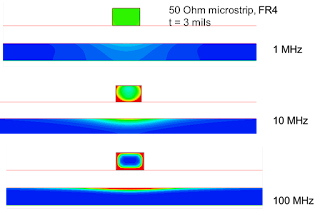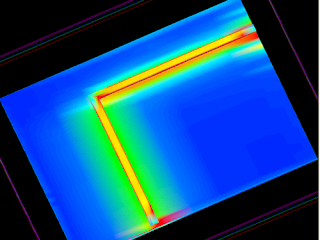## You need to test, we're here to help.

You need to test, we're here to help.

## 16 January 2023

### Signal Return Paths: A Signal and Power Integrity TutorialFigure 1. Current distribution in the signal and return conductors at three different frequencies. The current redistribution at higher frequency is driven by the currents taking filament paths with the lowest loop inductance.
The following is excerpted from Professor Eric Bogatin's article in the Signal Integrity Journal 2023 eBook, The Case for Split Ground Planes . Reprinted by permission of Signal Integrity Journal.

. . .

### Why Continuous Return Path Planes

The first step in engineering interconnects to reduce noise is to provide a continuous, low impedance return path to control the impedance, which controls reflection noise, and reduce the cross talk between signals that also share the same return conductor.

A wide, continuous ground plane adjacent to a signal trace will be the lowest cross talk configuration. Anything other than a wide plane means more cross talk between signal paths sharing this return conductor. This means, never add a split or gap in the return path. You would run the risk of a signal trace inadvertently crossing this discontinuity.

If a signal crosses over a split ground plane, there are two effects which compound each other. Crossing a split creates a higher impedance path for return currents that must cross the split and forces return currents from multiple signals to overlap through the same, higher impedance, common path.

This creates the trifecta of problems: reflections from the return path discontinuity, ground bounce from the higher inductance return path, and EMI from the difference in potential between the two regions of the planes in which the return currents flow. This is why it is never a good idea to add a split in the ground return plane. You take too big a risk of signals routed crossing this split.

However, there is one potential, minor problem a split ground plane solves, provided the split or gap in the return plane is ALWAYS parallel to the signal path, and return currents do not cross this gap. It is cross talk from resistive coupling.

### Where Return Currents Flow

The path return currents take in a ground plane depends on the routing of the signal paths. The signal and return paths cannot be considered separately. They are linked together. The path the currents take in a signal and return conductor are dictated by the path of lowest loop impedance. If there were a path the signal-return current took that was lower impedance, there would be a lower voltage drop along that path and currents would flow from the higher voltage paths to the lower voltage paths until all conductors, normal to the direction of propagation, are at an equipotential.

This means that of the multiple paths signal-return currents could take, the currents will flow in the path to minimize the loop impedance of all possible paths.

Usually, the signal trace is narrow and confines the signal current to a very specific path. The return current can flow in the adjacent ground plane anywhere, unconstrained except for the plane edges. It will take the path so that the loop impedance of the signal-return path is minimized. To first order, the impedance of the signal-return path is frequency dependent and related to:

Z = R + j𝛚L

In this equation,

Z is the loop impedance of the current loop path,

R is the series resistance of the loop and

L is the loop inductance of the path

Imagine the signal-return path currents as composed of continuous current filaments taking any path they can down the interconnect. The filaments that have the most current are those with the lowest loop impedance. The more current flowing down one of these filaments, the higher the voltage drop across this series impedance. This pushes more current into adjacent higher impedance filaments until the cross section of current distribution, balanced by the impedance of each filament and the amount of current in each filament, create an equipotential across the direction of propagation.

There will always be a frequency, above which the 𝛚L term dominates and the current paths are driven by the path of lowest loop inductance. This is the region we refer to as the skin depth region. The current redistribution for lowest loop inductance is what drives the skin depth effect.

The lowest impedance path is when the currents within the same conductor are farthest apart, to reduce the partial self-inductances, but are closest together between the signal and return paths, to increase the partial mutual inductances. This is illustrated in Figure 1, showing the current distributions in a simple microstrip at 1 MHz, 10 MHz and 100 MHz, simulated with Ansys Q2D.Figure 2. Current flow in the return path at 1 MHz, when the signal path changes.
This means that in the high frequency regime, the return currents are always flowing directly underneath the signal currents. The path underneath the signal currents is always the lowest loop inductance path. Any current filaments away from this path will have a higher impedance and a higher voltage drop and flow to the lower voltage filaments, directly under the signal.

As the signal conductor meanders over the surface of the ground plane, the return currents will follow right along under the signal path. Figure 2 shows an example of the return current distribution in a plane when the signal conductor changes direction, simulated for 1 MHz frequency components.